Ex 6.4

Chapter 6 Class 10 Triangles (Term 1)
Serial order wise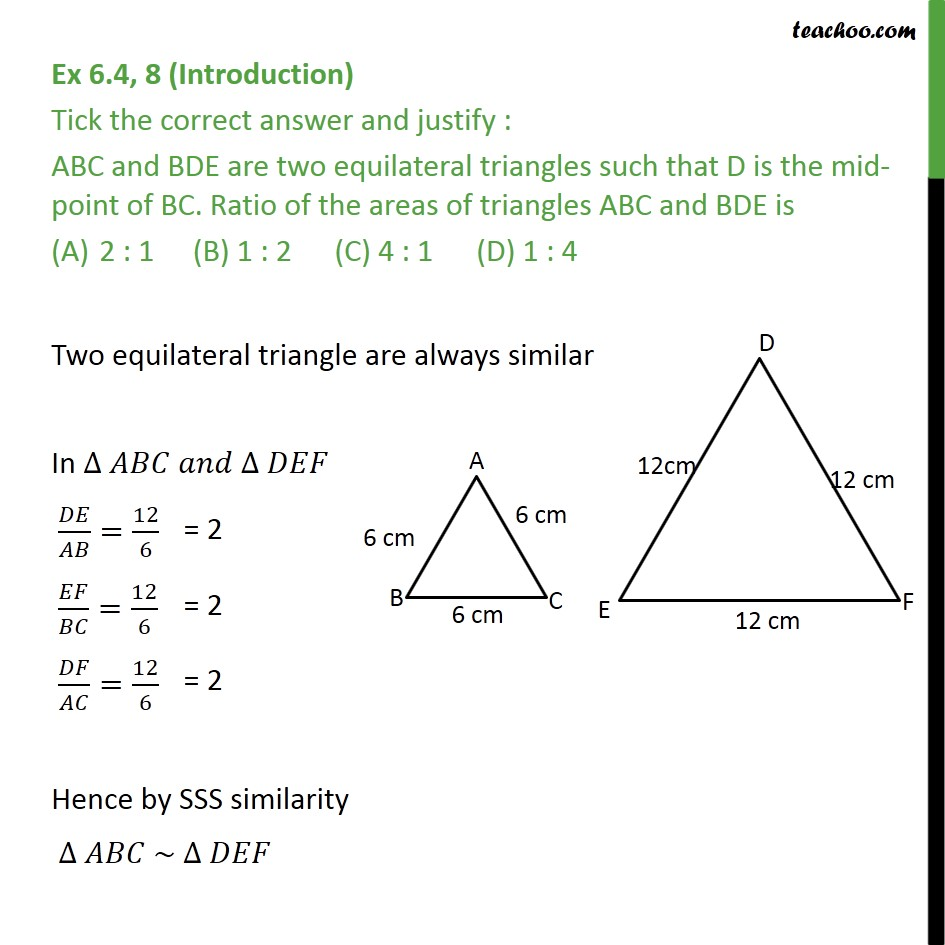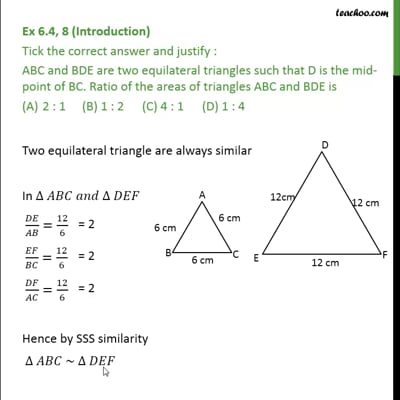This video is only available for Teachoo black users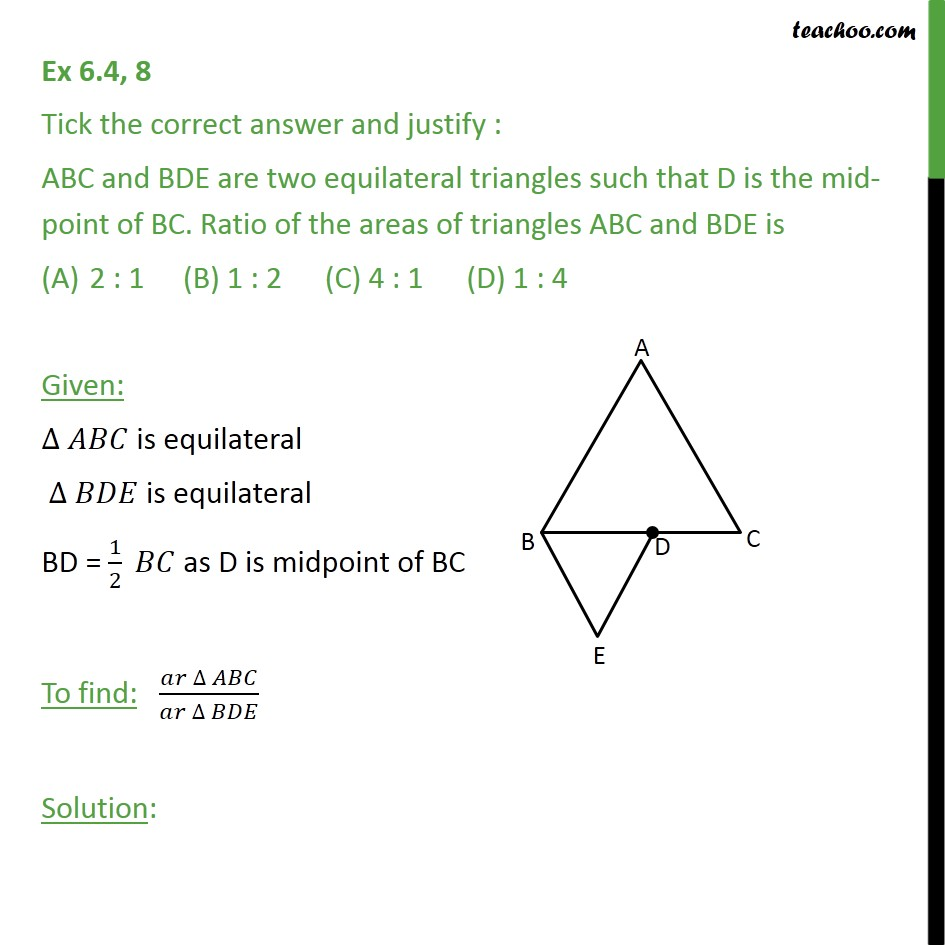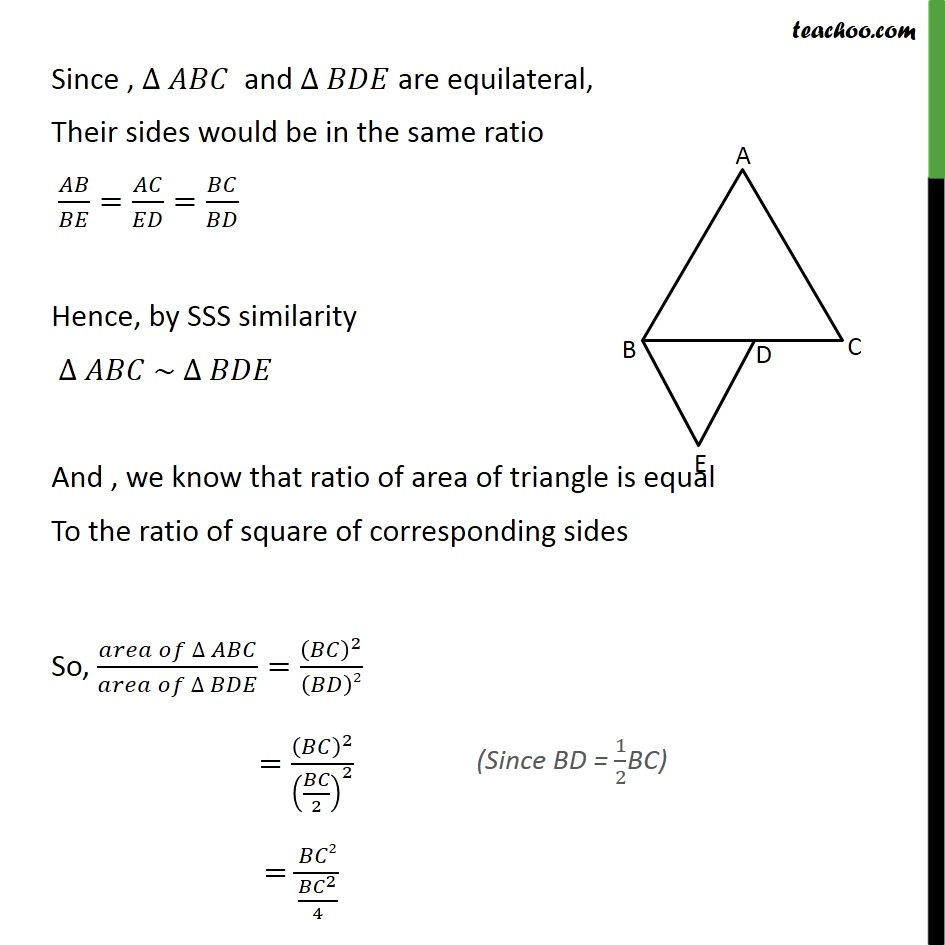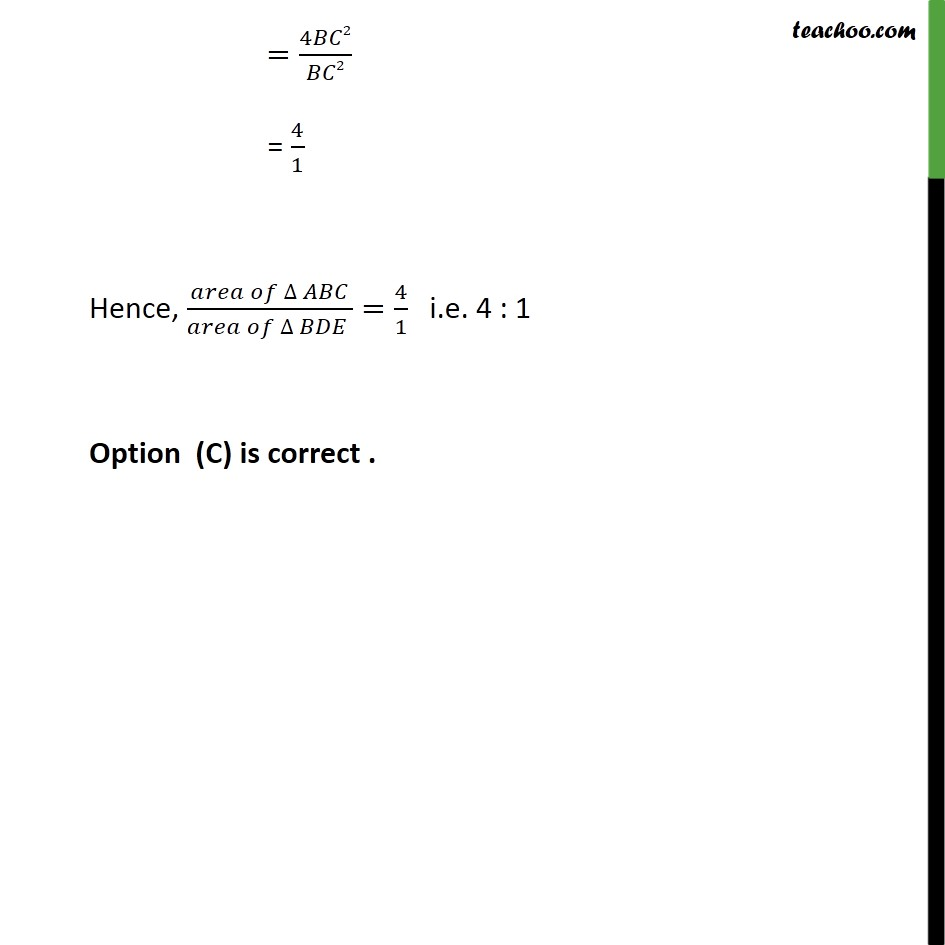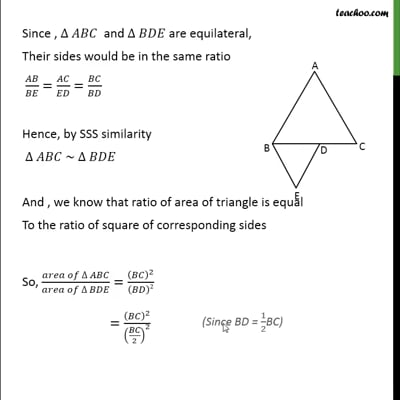This video is only available for Teachoo black users

### Transcript

Ex 6.4, 8 (Introduction) Tick the correct answer and justify : ABC and BDE are two equilateral triangles such that D is the mid-point of BC. Ratio of the areas of triangles ABC and BDE is 2 : 1 (B) 1 : 2 (C) 4 : 1 (D) 1 : 4 Two equilateral triangle are always similar In = 12 6 = 12 6 = 12 6 Hence by SSS similarity ~ Ex 6.4, 8 Tick the correct answer and justify : ABC and BDE are two equilateral triangles such that D is the mid-point of BC. Ratio of the areas of triangles ABC and BDE is 2 : 1 (B) 1 : 2 (C) 4 : 1 (D) 1 : 4 Given: is equilateral is equilateral BD = 1/2 as D is midpoint of BC To find: ( )/( ) Solution: Since , and are equilateral, Their sides would be in the same ratio / = / = / Hence, by SSS similarity ~ And , we know that ratio of area of triangle is equal To the ratio of square of corresponding sides So, ( )/( )=( )^2/( )2 =( )^2/( /2)^2 = 2/(( ^2)/4) =4 2/ 2 = 4/1 Hence, ( )/( )=4/1 i.e. 4 : 1 Option (C) is correct